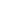Flat 50% off

Ends in

• JEE Main & Advanced
•• 11th
•• Mathematics
• Back to Doubt Clearing

## pls solve it The left hand derivative of $f(x) =[x]$sin $(\pi\times)$ at x=K k is an interger, is (a)  $(-1)^k(k-1)\pi$       (b) $(-1)^{k-1}(k-1)\pi$ (c) $(-1)^{k}k\pi$                  (d) $(-1)^{k-1} k\pi$

### Asked By Subhash kandepi

Updated Wed, 19 Dec 2018 11:34 am